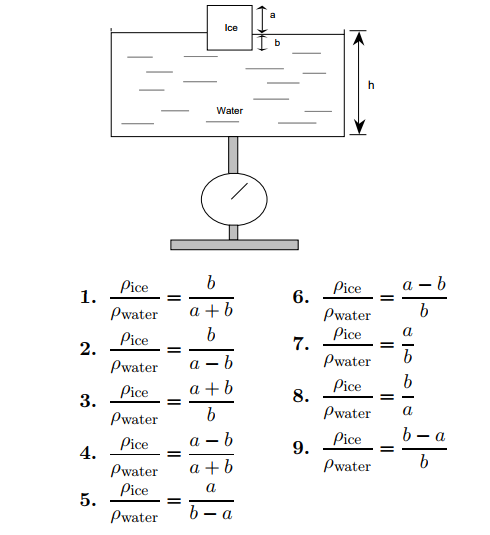# Problem: Consider a rectangular block of ice floating in water in an open vessel. Let  a denote the height of the ice block showing above the water surface, and b the height of the submerged part of the ice block. Given a, b, the density  ρwater of liquid water and the density  ρice of the ice, which relationship is correct?

###### FREE Expert Solution
84% (305 ratings)
###### Problem Details

Consider a rectangular block of ice floating in water in an open vessel. Let  a denote the height of the ice block showing above the water surface, and b the height of the submerged part of the ice block.

Given a, b, the density  ρwater of liquid water and the density  ρice of the ice, which relationship is correct?